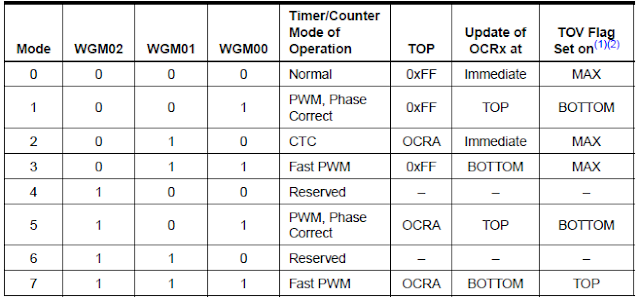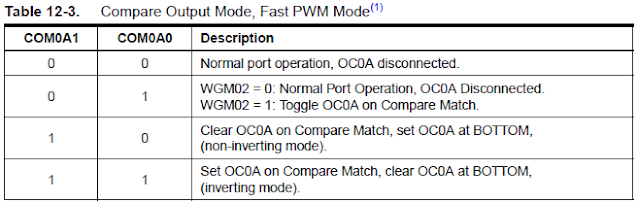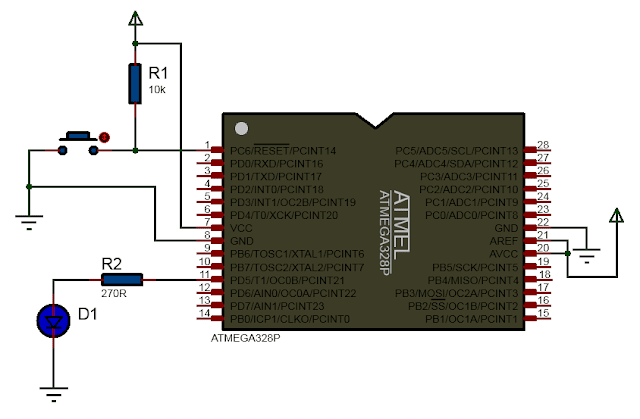# ATmega328P Fast PWM mode Programming Examples

ATmega328p micrcontroller Timer/Counter can be used to generate PWM signal. There are two types of PWM signal that can be generated which are Fast PWM and Phase Correct PWM. The Fast PWM mode is used for power regulation, rectification, and DAC applications. In this tutorial we will explain and illustrate Fast PWM mode for Timer/ Counter 0 with example codes.

For other ATmega328P modes of operation see the following:

- Normal Mode(see ATmega328p Timer Programming Examples)

- CTC mode(see Programming ATmega328p in CTC mode

- Phase Correct PWM mode(Phase Correct PWM with ATmega328P)

### Fast PWM mode

The Fast PWM mode is used to generate PWM signal at the output compare pins(either OC0A or OC0B depending upon which output compare unit A or B is used). It is called Fast because the generated PWM signal can have high frequency due to its single-slope operation.

### Modes of Fast PWM mode

There are two Fast PWM modes for Timer/Counter 0 unit. They are mode 3 and mode 7 which are selected using the waveform generation mode bits(WGM02, WGM01, WGM00). The following table shows the WGM bits combination for mode 3 and mode 7.The WGM02 bit is located in the TCCR0B register as shown below.The WGM01 and WGM00 bits are located in the TCCR0A register as shown below.

The difference between the mode 3 Fast PWM and mode 7 Fast PWM is the TOP value. For mode 3 the TOP value is is 0xFF whereas the the TOP value for mode 7 is OCRA. If mode 7 is used then we have to load count value into the OCR0A register. If mode 3 is used then we don't have to load the counter since the TOP value in this case is 0xFF. Thus in Fast PWM mode, we have to select either mode 3 or 7. Once selected the counter is started and the timer/counter starts counting from zero to TOP value and when TOP value is reached the counting is repeated from the bottom.

### Types of Fast PWM mode

There are two types of Fast PWM modes:

(a) non-inverting Fast PWM

(b) inverting Fast PWM

The Compare Output Mode bits COM0A1,COM0A0 for output at OC0A and COM0B1, COM0B0 bits for output at OC0B which are located in the TCCR0A register are used to configure the output as either non-inverting or inverting Fast PWM. The following table shows how to select the COM0A1, COM0A0 and COM0B1, COM0B0 bits for configuring non-inverting and inverting Fast PWM waveform.Following picture shows the timing diagram for Fast PWM which includes non-inverted and inverted PWM outputs.

### (a) Non-Inverting Fast PWM mode

To output Fast PWM signal we need to first decide which Fast PWM mode to use. This can be either mode 3 or mode 7. This choice in turn depends upon which output compare unit, A or B to use and we can have the following scenarios:

i) output fast pwm at OC0A pin only with mode 3

- the OCR0A register is loaded with duty cycle value

ii) output fast pwm at OC0B pin only with mode 3

- the OCR0B register is loaded with duty cycle value

iii) output fast pwm at both OC0A and OC0B pins with mode 3

- the OCR0A and OCR0B registers are loaded with duty cycle values

iii) output fast pwm at OC0B only with mode 7

- the OCR0B register is loaded with duty cycle value

- the OCR0A register is loaded with TOP value which must be equal or greater than the duty cycle

The Wave Generation Mode bits(WGM01 and WGM00) in TCCR0A register and WGM02 bit in the TCCR0B register are used to configure mode 3 or mode 7 Fast PWM mode. Once we have selected either mode 3 or mode 7 Fast PWM mode, we have to select either non-inverting or inverting Fast PWM mode. This is done by configuring the Compare Output Mode(COM0A1, COM0A0, COM0B1, COM0B0) bits.

### Non-Inverted Fast PWM Frequency & Duty Cycle

Non-Inverted Fast PWM Frequency

The Fast PWM frequency can be calculated by the following equation:

$$F_{w}=\frac{F_{osc}}{256N}$$           --------------->(1)

where, $$F_{w}$$ is the frequency of the generated PWM wave, $$F_{osc}$$ is the CPU frequency, N is the pre-scalar which can have value of 1, 8, 64,  256 and 1024.

For example, using equation (1), if $$F_{osc}$$ is 8MHz, pre-scalar N is 1 then the frequency of the generated Fast PWM signal is 31.25KHz.

The Clock Select(CS02, CS01 and CS00) bits located in the TCCR0B are used configure pre-scalar value(N). The following table shows the function of these CS bits.Thus the Fast PWM frequency can be set by using the CS bits.

Non-Inverted Fast PWM Duty Cycle

The duty cycle of the Fast PWM signal is calculated using the following formula.

$$OCR0 = \frac{256D}{100} - 1$$         ------------------------>(2)

where, D is the Duty cycle that range from 0% to 100%.

This duty cycle value is the count value that has to be loaded into the OCR0A(or OCR0B) register. For example if we want the PWM signal to have 75% duty cycle then using equation (2), the value for the OCR0A is 191.

We can use the online ATmega microcontroller Timer/Counter calculator to calculate the count value to be loaded into the OCR0A/OCR0B register. An example is shown below.

#### Non-Inverting Fast PWM mode 3 using OCR A unit

The steps to program ATmega328P for Fast PWM are:

(a) Make the OC0A pin(Port D Pin 6) an output pin

DDRD |= (1<<PD6);

(b) Load count value into the OCR0A register for duty cycle control

OCR0A = 191;

(c) Configure TCCR0A and TCCR0B register for (i) non-inverting, (ii) Fast PWM mode, (iii) Pre-scalar for frequency control

TCCR0A |= (1<<COM0A1) | (1<< WGM01) | (1<< WGM00);

TCCR0B |=  (1<<WGM02) | (1<<CS00);

The following is then the atmega328p fast pwm C program code for non-inverting Fast PWM with frequency of 31.25KHz and duty cycle of 75% using Timer/Counter0.

#ifndef F_CPU
#define F_CPU 8000000UL
#endif

#include <avr/io.h>

int main()
{
DDRD |= (1<<PD6);    //Fast PWM output at OC0A pin

OCR0A = 191;	// Duty cycle of 75%
TCCR0A |= (1<<COM0A1) | (1<<WGM01) | (1<<WGM00);	//Non-Inverting Fast PWM mode 3 using OCR A unit
TCCR0B |= (1<<CS00);	//No-Prescalar

while (1);

return 0;
}


The following schematic diagram shows Fast PWM output at the OC0A pin(port D pin 6).

The signal waveform graph shows duty cycle of 75% and Fourier graph shows the signal at 31.25KHz.

#### Non-Inverting Fast PWM mode 3 using OCR B unit

The following C program code will output non-inverting Fast PWM signal at the OC0B pin of ATmega328P.

#ifndef F_CPU
#define F_CPU 8000000UL
#endif

#include <avr/io.h>

int main()
{
DDRD |= (1<<PD5);    //Fast PWM output at OC0B pin

OCR0B = 191;	// Duty cycle of 75%
TCCR0A |= (1<<COM0B1) | (1<<WGM01) | (1<<WGM00);	//Non-Inverting Fast PWM mode 3 using OCR B unit
TCCR0B |= (1<<CS00);	//No-Prescalar

while (1);

return 0;
}


The following schematic diagram shows Fast PWM output at the OC0B pin(port D pin 5).

#### Non-Inverting Fast PWM mode 7 using OCR B unit as Output

In the mode 7 Fast PWM mode, the OCR0A register is used to hold the TOP value. Thus the output cannot be taken from the OC0A pin hence the output is only from the OC0B pin. Also in case of mode 7 the OCR0B register is used to set the duty cycle of the PWM. Important thing to remember is that the TOP value in OCR0A register must be less or equal to the Duty Cycle value in the OCR0B register.

The following C program illustrates how one can use the ATmega328P microcontroller to set the Fast PWM mode 7 in non-inverting mode with duty cycle of 75%(The duty cycle was calculated using the pwm duty cycle formula provided above), frequency of 31.25KHz, with TOP value of 200.

#ifndef F_CPU
#define F_CPU 8000000UL
#endif

#include <avr/io.h>

int main()
{
DDRD |= (1<<PD5);    //Fast PWM output at OC0B pin

OCR0A = 200;	// Top Value of 200(must be equal or greater than Duty Cycle)
OCR0B = 191;	// Duty cycle of 75%
TCCR0A |= (1<<COM0B1) | (1<<WGM01) | (1<<WGM00);	//Non-Inverting Fast PWM mode 7
TCCR0B |= (1<<WGM02) | (1<<CS00);	//No-Prescalar

while (1);

return 0;
}


The following circuit diagram shows Fast PWM mode 7 output at the OC0B pin.

### (b) Inverting PWM mode

Inverted Fast PWM Frequency

The frequency of the inverting PWM mode is the same as the frequency for non-inverting pwm mode. Thus the frequency of inverted PWM is given by equation (1) above and shown again below.

$$F_{w}=\frac{F_{osc}}{256N}$$

where, $$F_{w}$$ is the frequency of the generated wave and N is the pre-scalar which can have value of 1, 8, 64,  256 and 1024.

Inverted Fast PWM Duty Cycle

The duty cycle for the inverted Fast PWM signal is calculated using the following formula.

$$OCR0 = 255 - \frac{256D}{100}$$         ------------------------>(3)

where, D is the Duty cycle that range from 0% to 100%. For example for 75% duty cycle we get OCR0 to be 63.

We can use the online ATmega microcontroller Timer/Counter calculator to calculate the count value to be loaded into the OCR0A/OCR0B register. An example is shown below.

#### Inverted Fast PWM mode 3 using OCR A unit

Below is program code to generate inverted Fast PWM signal at OC0A pin with duty cycle of 75% which is loaded into the OCR0A register and frequency of 31.25KHz. The duty cycle was calculated using the pwm duty cycle formula provided above. To set the inverted mode we have to set both the COM0A1 and COM0A0 bits in the TCCR0A register.

#ifndef F_CPU
#define F_CPU 8000000UL
#endif

#include <avr/io.h>

int main()
{
DDRD |= (1<<PD6);    //Fast PWM output at OC0A pin

OCR0A = 63;	// Duty cycle of 75%
TCCR0A |= (1<<COM0A1) | (1<<COM0A0) | (1<<WGM01) | (1<<WGM00);	//Inverting Fast PWM mode 3
TCCR0B |= (1<<CS00);	//No-Prescalar

while (1);

return 0;
}


The following circuit diagram shows Fast PWM output at the OC0A pin.

#### Inverted Fast PWM mode 3 using OCR B unit

Below is program C code to generate inverted Fast PWM signal at OC0B pin with duty cycle of 75% which is loaded into the OCR0B register(pwm duty cycle formula is provided above) and frequency of 31.25KHz. To use inverted mode we have to set both the COM0B1 and COM0B0 bits in the TCCR0A register.

#ifndef F_CPU
#define F_CPU 8000000UL
#endif

#include <avr/io.h>

int main()
{
DDRD |= (1<<PD5);    //Fast PWM output at OC0B pin

OCR0A = 63;	// Duty cycle of 75%
TCCR0A |= (1<<COM0B1) | (1<<COM0B0) | (1<<WGM01) | (1<<WGM00);	//Inverting Fast PWM mode 3
TCCR0B |= (1<<CS00);	//No-Prescalar

while (1);

return 0;
}


The following circuit diagram shows inverted Fast PWM output at the OC0B pin.#### Inverted Fast PWM mode 7

For generating inverted Fast PWM in mode 7, we have to use the OCR0A for TOP value for counting. In this case we have loaded OCR0A top value of 200. This top value must be greater than the duty cycle value 191 for 75% duty cycle which is loaded into the OCR0B register. The duty cycle was calculated using the pwm duty cycle formula provided above. In case of mode 7, the output PWM signal is taken from OC0B pin. To use mode 7 we must set all the wave generation mode bits WGM02 located in TCCR0B, WGM01 and WGM00 which are located in TCCR0A register.

Following is the C program code for generating inverted Fast PWM signal in mode 7.

#ifndef F_CPU
#define F_CPU 8000000UL
#endif

#include <avr/io.h>

int main()
{
DDRD |= (1<<PD5);    //Fast PWM output at OC0A pin
OCR0A = 200;	// Top Value of 200(must be equal or greater than Duty Cycle)
OCR0B = 63;	// Duty cycle of 75%

TCCR0A |= (1<<COM0B1) | (1<<COM0B0) | (1<<WGM01) | (1<<WGM00);	//Inverting Fast PWM mode 7
TCCR0B |= (1<<WGM02) | (1<<CS00);	//No-Prescalar

while (1);

return 0;
}

You need to compile and upload the above code into the microcontroller using atmega328p programming software. If you are having difficulty in deploying and checking out whether it is working or not you can try first simple atmega328p led blink program.

So in this tutorial on generating PWM signals using timers in the atmega328p chip, we have illustrated the Fast PWM mode with example code. In the next tutorial we will show how to use the Phase Correct PWM with ATmega328P.

For other tutorials on PWM see the followings:

PWM - Programming Arduino using Matlab

DC motor Speed control with Potentiometer and PWM using Arduino

Speed control of DC motor with PWM using Arduino